One of the sufficiency criteria for the convergence of a Fourier series at a point. Let a functionhave period, be Lebesgue integrable over the interval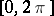and, on putting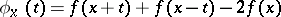, let it satisfy at the pointthe conditions: 1)as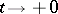; 2) the function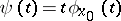is of finite variationon the interval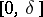,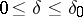, whereis some fixed number; and 3)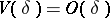as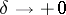. Then the Fourier series ofatconverges to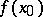(cf. ). Young's criterion is stronger than the Jordan criterion. It was established by W.H. Young .